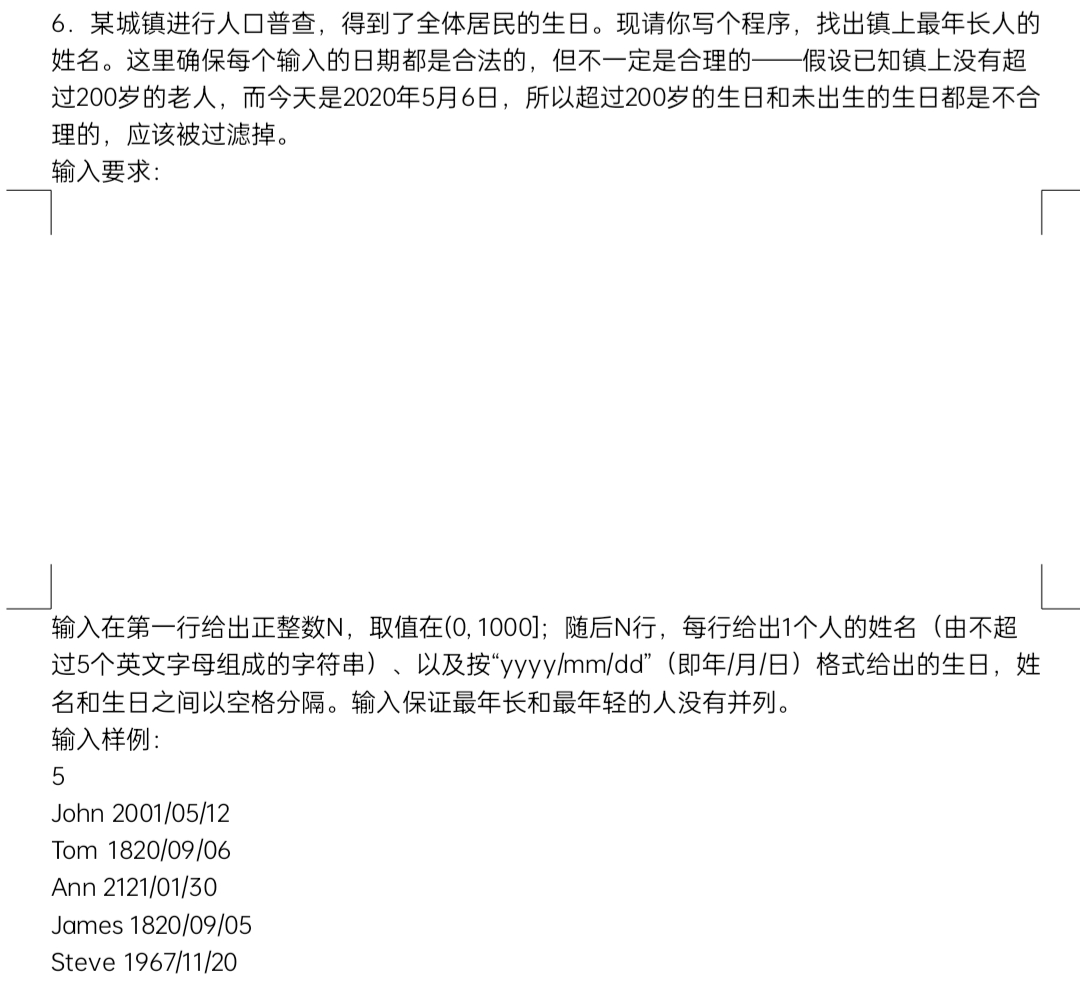2021-11-19 23:34

一道python问题，求解答！• 写回答
• 好问题 提建议
• 追加酬金
• 关注问题
• 收藏
• 邀请回答

1条回答默认 最新

•已采纳

persons = {}
name, date = person.split() # 将字符串分割成名字和出生日期
y, m, d = map(int, date.split('/')) # 将出生日期分割成年月日并转成数字
if y>2020 or (y==2020 and m > 5) or (y==2020 and m==5 and d>6): # 未出生
pass
elif y<1820 or (y==1820 and m < 5) or (y==1820 and m==5 and d<6):   # 超过200
pass
else:
persons[name] = [y, m, d]

if __name__ == '__main__':
n = int(input('输入人口数量[1-1000]：'))
if n<=0 or n>1000:
print('输入的人口数量不合理')

for _ in range(n):